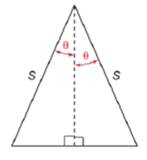Chapter 11.2, Problem 42EElementary Geometry For College St...

7th Edition
Alexander + 2 others
ISBN: 9781337614085

Solutions

Chapter
SectionElementary Geometry For College St...

7th Edition
Alexander + 2 others
ISBN: 9781337614085
Textbook Problem

For Exercise 42 and 43, use the drawing and the formula from Exercise 41.Find the area of an isosceles triangle for which s = 10.6     c m and the measure of the vertex angle is 46 ∘ .

To determine

To find:

To the area of an isosceles triangle for which s=10.6cm and the measure of the vertex angle is 46 by using the following figure,Explanation

Consider the following figure,

The area of the triangle is given below,

A=s2sinθcosθ

Given that s=10.6cm and the measure of the vertex angle is 46.

From the above figure shows that the measure of the vertex angle is

2θ=46θ=462θ=23

Substitute the values in the area formula to get the following,

A=(10.6)2sin23cos23

Use the graphing calculator to find the following,

sin23=0

Still sussing out bartleby?

Check out a sample textbook solution.

See a sample solution

The Solution to Your Study Problems

Bartleby provides explanations to thousands of textbook problems written by our experts, many with advanced degrees!

Get Started

Evaluate the integral. /6sind

Single Variable Calculus: Early Transcendentals, Volume I

In Exercises 73-80, find the indicated limits, if they exist. 77. limxx4+1x31

Applied Calculus for the Managerial, Life, and Social Sciences: A Brief Approach

Under what circumstances is a t statistic used instead of a z-score for a hypothesis test?

Essentials of Statistics for The Behavioral Sciences (MindTap Course List)

In Problems 20 and 21, find any horizontal asymptotes and any vertical asymptotes. 21.

Mathematical Applications for the Management, Life, and Social Sciences

Explain the terms (a) scatter diagram (b) least-squares line.

Finite Mathematics for the Managerial, Life, and Social Sciences

The polar coordinates of the point P in the figure at the right are:

Study Guide for Stewart's Multivariable Calculus, 8th

The polar equation of the conic with eccentricity 3 and directrix x = −7 is:

Study Guide for Stewart's Single Variable Calculus: Early Transcendentals, 8th